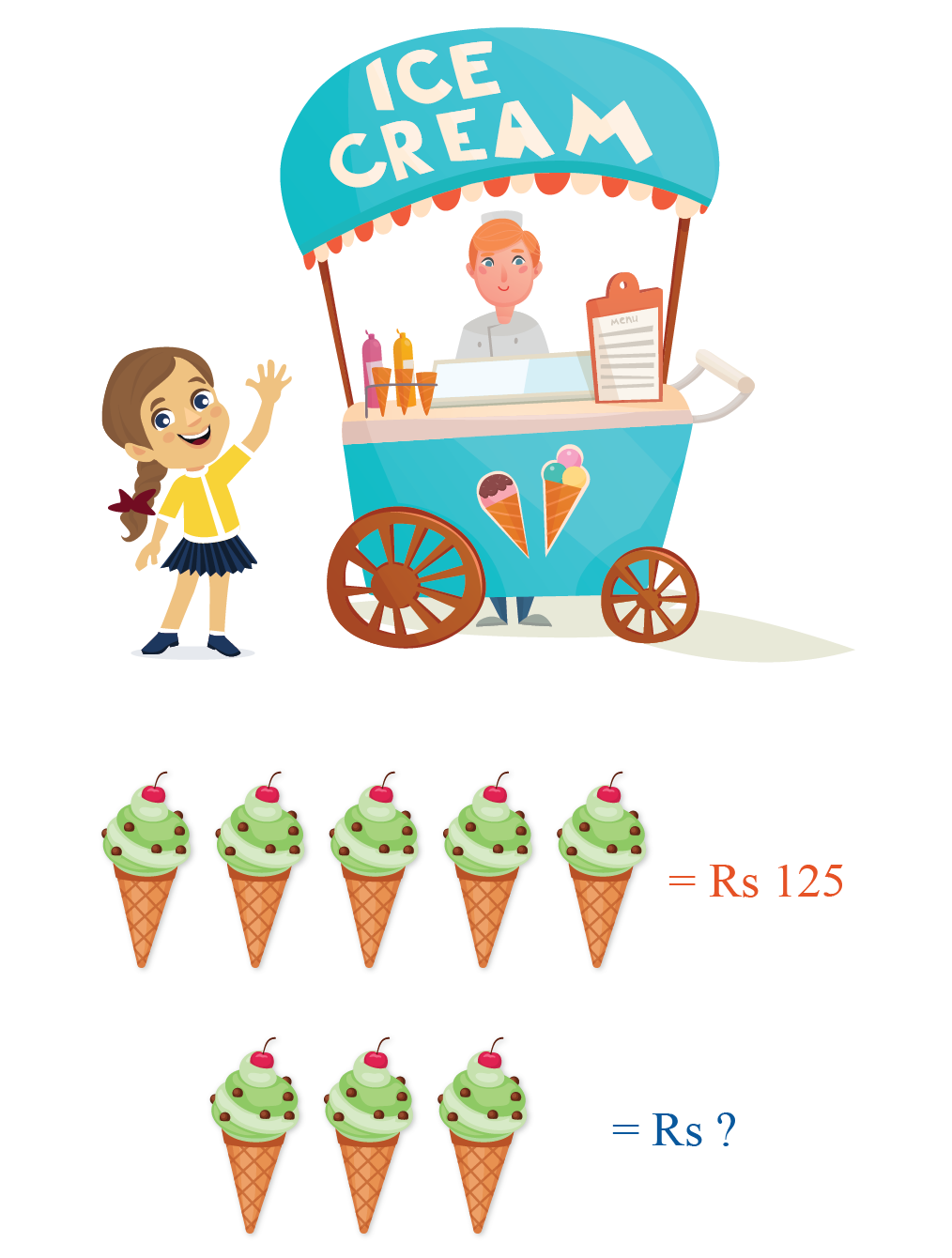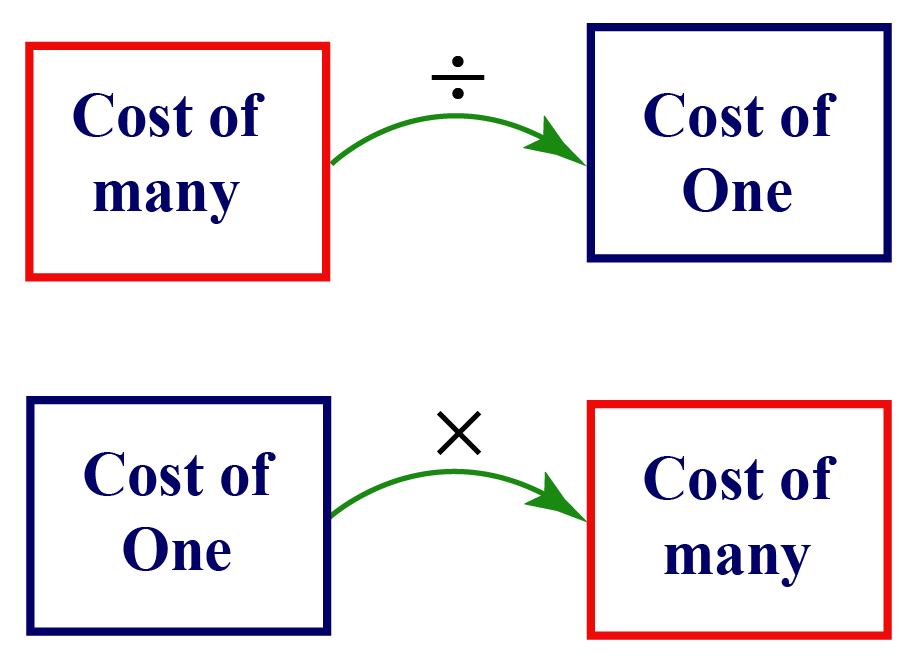# Unitary Method

Let's explore the world of unitary method with real life examples in this mini lesson. We will learn about definition of unitary method, its types and stepwise solutions to the problems on it. So, let's get going!

Hey kids! Do you get confused at times when you need to make payment on buying some items?

Want to learn a method that makes your shopping experience better by doing the correct transaction and not get cheated on the prices at any time?

Here we have unitary method to help you out! It's very useful method when we go to a shop to buy books and other stationaries.Unitary method is a method where we find the value of a single unit from the value of multiple units and the value of multiple units from the value of a single unit.

## Lesson Plan

 1 What is Unitary Method? 2 Solved Examples Based on Unitary Method 3 Interactive Questions 4 FREE Downloadable Resources on Unitary Method

## Free PDFs for Offline Revision

Get your copy of Unitary Method E-book along with Worksheets and Tips and Tricks PDFs for Free!

## What is Unitary Method?

Let's recap the definition.

"Unitary method is a method where we find the value of a single unit from the value of multiple units and the value of multiple units from the value of a single unit."

Here is  a situation to understand this method.

Ria went to an ice-cream parlour and bought $$5$$ ice-creams.

She paid $$\text{Rs.}\;125$$ to the shopkeeper.

Next day she again goes to same parlour and orders $$3$$ icecreams. So, how much will she be paying for $$3$$ ice-creams?This may seem hard to calculate! However, we can solve this problem using the unitary method.

### Steps :

First, let us make a note of the information we have.

There are $$5$$ ice-creams.

$$5$$ ice-creams cost $$\text{Rs.}\;125$$.

STEP 1:

Let’s find the cost of $$1$$ ice-cream.

In order to do that, divide the total cost of ice-creams by the total number of ice-creams.

The cost of 1 ice-cream:

\begin{align}&=\frac{\text{Total cost of ice-creams}}{\text{Total number of ice-creams}}\\&=\frac{125}{5}\\&=25\end{align}

Therefore, the cost of $$1$$ ice-cream is $$\text{Rs.}\;25$$.

STEP 2:

To find the cost of $$3$$ ice-creams, multiply the cost of $$1$$ ice-cream with the number of ice-creams.

Cost of 3 ice-creams:

\begin{align} &\!= \!\text{Cost of 1 ice-cream} \! \times \! \text{Number of ice-creams} \\ & \!=\! 25 \! \times \! 3 \\ & \!=\! 75 \\ \end{align}

Finally, we have the cost of $$3$$ ice-creams i.e. $$\text{Rs.}\;75$$.

In unitary method, the value of many things is given and we need to either find the value of more or less things.

In order to do that, we must first find the value of one thing by division and then find the value of more or less things by multiplication.Unitary Method- Worksheet
UNitary MEthod Worksheet
Unitary Method- Tips and Tricks
Tips and Tricks
Unitary Method- Ebook
Ebook
More Important Topics
Numbers
Algebra
Geometry
Measurement
Money
Data
Trigonometry
Calculus